> 文档中心 > 基于到达时间差（TDOA）的室内定位（/无线传感器网络定位）——极大似然估计ML

# 基于到达时间差（TDOA）的室内定位（/无线传感器网络定位）——极大似然估计ML

WX: ZB823618313

### 基于到达时间差（TDOA）的室内定位（/无线传感器网络定位）——极大似然估计ML

• 基于到达时间差（TDOA）的室内定位（/无线传感器网络定位）——极大似然估计ML
• 1. 室内定位/无线传感器网络定位技术
• 1.1. 室内定位/无线传感器网络定位分类
• 1.2. 室内定位/无线传感器网络定位特点
• 1.3. 室内定位/无线传感器网络定位技术分类
• 1.4. 室内定位/无线传感器网络定位技术比较
• 2. TDOA定位介绍
• 2.1 TDOA定位原理
• 2.2 TDOA拓扑图
• 2.3 TDOA与TOA对比
• 2.4 TDOA定位测量
• 3. TOA定位算法概述
• 4. ML最大似然估计TOA定位
• 4.1. 问题描述
• 4.2. ML估计
• 5. 仿真结果 1
• 5.1. 仿真场景
• 5.2. 定位误差RMSE(蒙特卡洛实现)

## 1. 室内定位/无线传感器网络定位技术

### 1.3. 室内定位/无线传感器网络定位技术分类

• 到达角度技术(AOA)
• 达到时间技术(TOA)
• 到达时间差技术(TDOA)

### 1.4. 室内定位/无线传感器网络定位技术比较

AOA 中、高 LOS 角度 只需要两个接收器。 不需要同步时间 需要天线阵列、价格昂贵
TOA LOS 距离 精度高 需要三个接收器、发射源和目标均时间同步
TDOA LOS 距离差 精度高，仅需要同步发射源的始终 LOS假设

## 2. TDOA定位介绍

### 2.1 TDOA定位原理

1. 第1种形式是利用移动台到达2个基站的时间TOA，取其差值来获得，这时仍需要基站时间的严格同步，但是当两基站间移动信道传输特性相似时，可减少由多径效应带来的误差，

2. 第2种形式是将一个移动台接收到的信号与另一个移动台接收到的信号进行相关运算，从而得到TDOA的值，这种算法可以在基站和移动台不同步时，估计出TDOA的值，由于实际应用中，往往很难做到基站与移动台的同步，所以利用相关估计得到TDOA值，再进行定位计算能获得较高精度。对于蜂窝网中的移动台定位而言，TDOA更具有实际意义，这种方法对网络的要求相对较低，并且定位精度较高，已经成为研究的热点。

### 2.2 TDOA拓扑图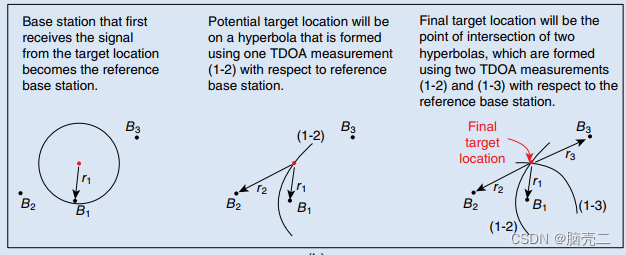### 2.3 TDOA与TOA对比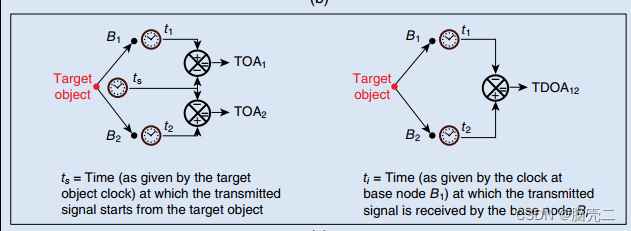### 2.4 TDOA定位测量

t T D O A =ti −t1 ,   i = 2 , 3 , ⋯   , N t_{TDOA}=t_i-t_1,~i=2,3, \cdots,N

TDOA 定位方法，主要是根据测量接收信号在基站和移动台之间的到达时间，然后转换为距离，从而进行定位。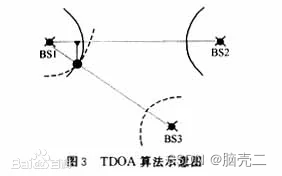di = ( x − x i ) 2+ ( y − y i ) 2 d_i=\sqrt{(x-x_i)^2+(y-y_i)^2}

t T D O A , i =ti −t1 ,   i = 2 , 3 , ⋯   , N t_{TDOA,i}=t_i-t_1,~i=2,3, \cdots,N

r T D O A , i = d i − d 1 + n i = ( x − x i ) 2 + ( y − y i ) 2 − ( x − x 1 ) 2 + ( y − y 1 ) 2 + n i ,   i = 2 , ⋯   , N \begin{aligned} r_{TDOA,i}&=d_i-d_1+n_i\\ &=\sqrt{(x-x_i)^2+(y-y_i)^2}-\sqrt{(x-x_1)^2+(y-y_1)^2}+n_i, ~ i=2,\cdots,N \end{aligned}

## 3. TOA定位算法概述

1-参数估计算法主要解决非移动目标（如传感器节点、发射源等）的定位问题，主要包括：
ML最大似然估计
LS最小二乘估计
WLS加权最小二乘估计
NLS非线性最小二乘估计

**注意：**这类方法也可以解决移动目标的室内外定位问题。

%%%%%%%%%%%%%%%%%%%%%%%%%%%%%%%%%%%%%%%%%%%%%%%%%

%%%%%%%%%%%%%%%%%%%%%%%%%%%%%%%%%%%%%%%%%%%%%%%%%

2- 滤波方法主要解决移动目标（如智能小车、移动机器人、移动终端等）的室内外定位问题，主要包括：

Divided difference filter DDF

… …

## 4. ML最大似然估计TOA定位

### 4.1. 问题描述

t T D O A , i =ti −t1 ,   i = 2 , 3 , ⋯   , N t_{TDOA,i}=t_i-t_1,~i=2,3, \cdots,N

r T D O A , i = d i − d 1 + n i = ( x − x i ) 2 + ( y − y i ) 2 − ( x − x 1 ) 2 + ( y − y 1 ) 2 + n i ,   i = 2 , ⋯   , N \begin{aligned} r_{TDOA,i}&=d_i-d_1+n_i\\ &=\sqrt{(x-x_i)^2+(y-y_i)^2}-\sqrt{(x-x_1)^2+(y-y_1)^2}+n_i, ~ i=2,\cdots,N \end{aligned}

### 4.2. ML估计

P ( α ∣ x ) =∏ i = 1 N 1 2 π σ i e −( α i − h ( x ) ) 2 2 σ i 2 P(\mathbf{\alpha}|\mathbf{x})=\prod_{i=1}^N\frac{1}{\sqrt{2\pi}\sigma_i}e^{-\frac{(\alpha_i-h(\mathbf{x}))^2}{2\sigma_i^2}}

h ( x ) = ( x − x i ) 2+ ( y − y i ) 2 − ( x − x 1 ) 2+ ( y − y 1 ) 2 h(\mathbf{x})=\sqrt{(x-x_i)^2+(y-y_i)^2}-\sqrt{(x-x_1)^2+(y-y_1)^2}

L ( x ) = c +∑ i = 1 N −1 2 σ i 2 (αi − h ( x ))2 L(\mathbf{x})=c+ \sum_{i=1}^N-\frac{1}{2\sigma_i^2}(\alpha_i-h(\mathbf{x}))^2

x ^ = arg ⁡ max ⁡ L ( x ) = arg ⁡ min ⁡ ∑ i = 1 N 1 2 σ i 2 ( α i − h ( x ) ) 2 \begin{aligned} \hat{\mathbf{x}}&=\arg \max L(\mathbf{x})\\ &=\arg \min \sum_{i=1}^N\frac{1}{2\sigma_i^2}(\alpha_i-h(\mathbf{x}))^2 \end{aligned}

## 5. 仿真结果 1

### 5.1. 仿真场景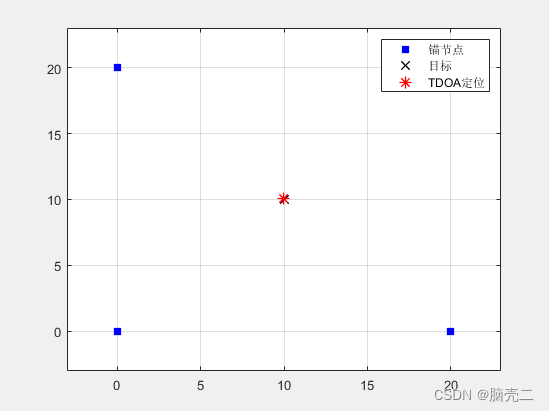TDOA定位结果:10.006710.0299

### 5.2. 定位误差RMSE(蒙特卡洛实现)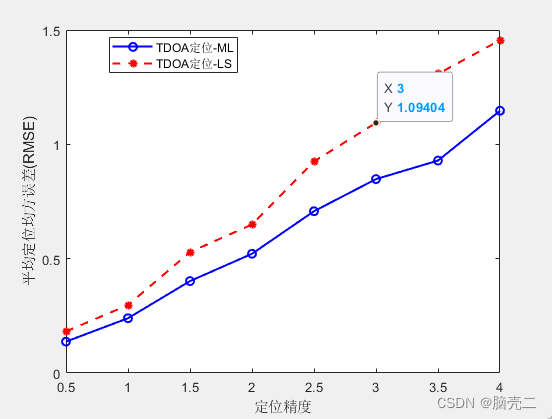TDOA定位结果:

Target_position_ML =    10.0202    9.8870    9.9408   10.7513    9.0884    9.8172    9.9751    9.4494   10.0533   10.2017    9.7053    9.9880   10.1940    9.7524   11.1078   10.4629

*原创不易，路过的各位大佬请点个赞